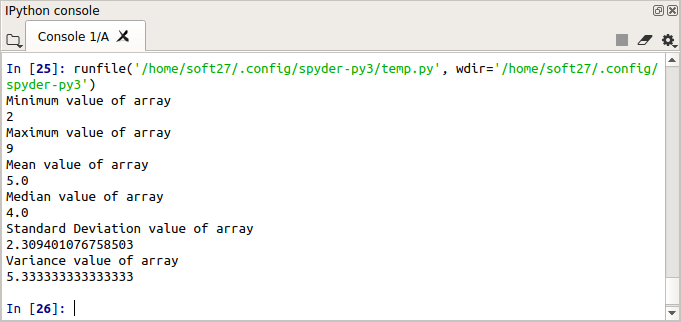• #5, First Floor, 4th Street Dr. Subbarayan Nagar Kodambakkam, Chennai-600 024 Landmark : Samiyar Madam
• pro@slogix.in
• +91- 81240 01111

### How to use statistical functions from python NumPy module?

###### Description

To perform some statistical operations like mean, median,standard deviation, minimum, maximum and variance using python NumPy module.

###### Process

NumPy stands for Numerical Python.

NumPy having some in build methods like mean(),np.median(),np.std()and np.var() to calculating the basic statistics functions.

We can create N-dimensional array using NumPy module.

Its majorly for numeric operations.

###### Sample Code

#import numpy
import numpy as np

#sample array
array = np.array([[3,7,5],[8,4,3],[2,4,9]])

#minimum value
print(“Minimum value of array”)
print(np.amin(array))

#Maximum value
print(“Maximum value of array”)
print(np.amax(array))

#calculating mean
print(“Mean value of array”)
print(np.mean(array))

#calculating median
print(“Median value of array”)
print(np.median(array))

#calculating standard deviation
print(“Standard Deviation value of array”)
print(np.std(array))

#calculating variance
print(“Variance value of array”)
print(np.var(array))

###### Screenshots